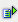The function fact (int) calculates the factorial of a number.

Syntax: =fact(int)Example =fact(0) is 0 =fact(1) is 1 =fact(2) is 2 =fact(3) is 6 =fact(4) is 24 =fact(5) is 120